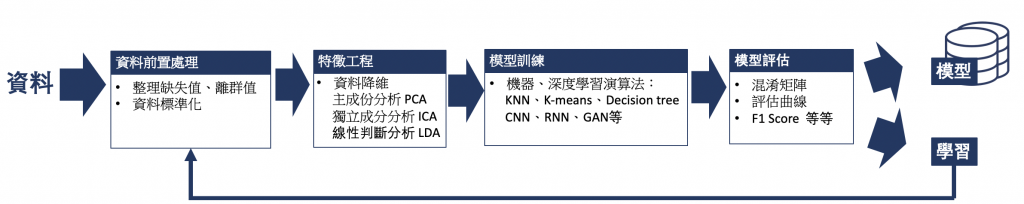#DAY 2
0

## Day2 機器學習介紹

``````# isnull()當缺失值位置為True,非缺失值位置為False的DataFrame
dataframe = df.isnull()
# 刪除缺失的行
df_nan_row = df.dropna(axis=0)
# 刪除缺失的列(一般不因為某列有缺失值就刪除列,因為列常代表某指標,但可能會因為該列近全部的資料皆為空值時，回刪除該列資料)
df_nan_col = df.dropna(axis=1)
# 以平均值替代缺失值
df1_fill_lack2 = df1.fillna(df1.mean())

# drop_duplicates()直接對重複資料（行）進行刪除
df_isdup = df.duplicated()
``````## 學習資源

1. 實戰機器學習-以學習演算企業資料 李博 著